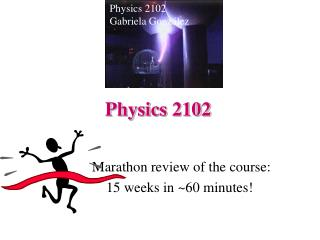DownloadDownload PresentationPhysics 2102

# Physics 2102

Download Presentation## Physics 2102

- - - - - - - - - - - - - - - - - - - - - - - - - - - E N D - - - - - - - - - - - - - - - - - - - - - - - - - - -
##### Presentation Transcript

1. Physics 2102 Gabriela González Physics 2102 Marathon review of the course: 15 weeks in ~60 minutes!

2. Overview • Fields: electric & magnetic • electric and magnetic forces on electric charges • potential energy, electric potential, work (for electric fields), electric and magnetic energy densities • fundamental laws on how fields are produced:Maxwell’s equations! • Circuits & components: • Capacitors, resistors, inductors, batteries • Circuits: circuits with R and batteries, RC, LR, LC. • Waves : • Speed, frequency, wavelength, polarization, intensity • Wave optics: interference

3. Electric Field • Forces due to electric fields: F=qE, Coulomb’s Law • Electric field due to: • single point charge, several point charges • Electric dipoles: field produced by a dipole, electric torque on a dipole • charged lines: straight lines, arcs • surface charges: conducting and insulating planes, disks, surfaces of conductors • volume charges: insulating spheres, conductors (E=0 inside). • Electric flux, Gauss’ law, applied to spherical, cylindrical, plane symmetry • Electric potential of a single charge, of several charges, of distributed charges. • Work, potential energy Gauss’ law!

4. Electric fields

5. Magnetic Fields • Force exerted by a magnetic field on a charge, on a current. • Magnetic field lines: always closed! • Magnetic fields created by currents:wires, loops, solenoids (Biot-Savart’s law, Ampere’s law) • Magnetic dipoles: field produced by a dipole, torque on a dipole

6. Magnetic fields

7. Induced fields • Changing magnetic flux creates an induced electric field (and a current if there is a wire!): Faraday’s law • Changing electric flux creates an induced magnetic field: Ampere-Maxwell’s law, displacement current

8. Induced fields

9. Maxwell’s equations Plus:

10. V=iR, Q=CV, E=-Ldi/dt Resistivity: E=Jr, R=rL/A Parallel plate, spherical, cylindrical capacitors Capacitors with dielectrics Resistors, capacitors in series and in parallel Power delivered by a battery: P=iE Energy dissipated by a resistor: P=i2 R=V2/R Energy stored in capacitors, inductors (energy stored in electric, magnetic fields) Ideal and real batteries (internal resistance) Resistors, Capacitors, Inductors V=iR Q = CV

11. DC Circuits • Single and multiloop circuits: • Junction rule for current • Loop rule for potential difference • RC/RL circuits: • time constant: t=RC, L/R • charging/discharging • POTENTIAL across capacitor CANNOT CHANGE SUDDENLY! • CURRENT in inductor CANNOT CHANGE SUDDENLY !

12. DC circuits Plus: resistors and capacitors in series and in parallel

13. AC Circuits • LC Oscillations: • careful about difference between frequency & angular frequency! • Physical understanding of stages in LC cycle • RLC circuits: energy is dissipated in the resistor.

14. Circuits, circuit elements

15. Electromagnetic waves Wave propagating in x direction: E=Em sin(kx-wt) j B=Bm sin(kx-wt) k c=Em/Bm=(m0e0)-1/2: speed of light in vacuum S=ExB/m0=(Em2/m0c)sin2(kx-wt) i : Poynting vector I= Em2/2m0c: intensity, or power per unit area Spherical waves: I=Ps/4pr2 Radiation pressure: F/A=I/c (absorption), F/A=2I/c (reflection) Polarizers: I=I0/2 (unpolarized light), I=I0cos2q (polarized light)

16. Wave Optics • Refraction: l= l0/n, v=c/n, n2sinq2=n1sinq1 v1sinq2=v2sinq1 • Two-beam Interference due to difference in phase : DF/(2p)=DL/l • DL=ml (constructive), DL=(m+1/2)l (destructive) • Coherent light through a double slit produces fringes: dsinq=ml (bright), dsinq=(m+1/2)l (dark), fringe spacing Dx=Ll/d

17. EM waves

18. Overview • Fields: electric & magnetic • electric and magnetic forces on electric charges • potential energy, electric potential, work (for electric fields), electric and magnetic energy densities • fundamental laws on how fields are produced:Maxwell’s equations! • Circuits & components: • Capacitors, resistors, inductors, batteries • Circuits: circuits with R and batteries, RC, LR, LC. • Waves : • Speed, frequency, wavelength, polarization, intensity • Wave optics: interference

19. Summary • Think about and understand basic concepts! • Look at your past exams and quizzes: why didn’t you get 100%? Predict your problems in the final exam! • Study the equation sheet, invent a problem for each formula. • Read all lecture slides, review hwk problems and problems in class. • Practice with a couple of past exams: timing is important! • Save time to eat lunch and relax the hour before the exam. • Enjoy the summer!